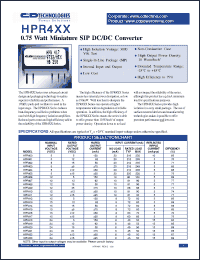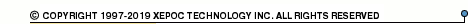More than4 307 195 components listedHPR4 series datasheets. Manufacturer: CANDD.

 HPR400 0.75 Watt miniature DC/DC converter. Nom.input voltage 5VDC, rated output voltage 5VDC, rated output current 150mA. in 7-pin SIP package. Operational temperature range from -25°C to 85°C. Datasheet*) HPR401 0.75 Watt miniature DC/DC converter. Nom.input voltage 5VDC, rated output voltage 12VDC, rated output current 62mA. in 7-pin SIP package. Operational temperature range from -25°C to 85°C. Datasheet*) HPR402 0.75 Watt miniature DC/DC converter. Nom.input voltage 5VDC, rated output voltage 15VDC, rated output current 50mA. in 7-pin SIP package. Operational temperature range from -25°C to 85°C. Datasheet*) HPR403 0.75 Watt miniature DC/DC converter. Nom.input voltage 5VDC, rated output voltage +-5VDC, rated output current +-75mA. in 7-pin SIP package. Operational temperature range from -25°C to 85°C. Datasheet*) HPR404 0.75 Watt miniature DC/DC converter. Nom.input voltage 5VDC, rated output voltage +-12VDC, rated output current +-30mA. in 7-pin SIP package. Operational temperature range from -25°C to 85°C. Datasheet*) HPR405 0.75 Watt miniature DC/DC converter. Nom.input voltage 5VDC, rated output voltage +-15VDC, rated output current +-25mA. in 7-pin SIP package. Operational temperature range from -25°C to 85°C. Datasheet*) HPR406 0.75 Watt miniature DC/DC converter. Nom.input voltage 12VDC, rated output voltage 5VDC, rated output current 150mA. in 7-pin SIP package. Operational temperature range from -25°C to 85°C. Datasheet*) HPR407 0.75 Watt miniature DC/DC converter. Nom.input voltage 12VDC, rated output voltage 12VDC, rated output current 62mA. in 7-pin SIP package. Operational temperature range from -25°C to 85°C. Datasheet*) HPR408 0.75 Watt miniature DC/DC converter. Nom.input voltage 12VDC, rated output voltage 15VDC, rated output current 50mA. in 7-pin SIP package. Operational temperature range from -25°C to 85°C. Datasheet*) HPR409 0.75 Watt miniature DC/DC converter. Nom.input voltage 12VDC, rated output voltage +-5VDC, rated output current +-75mA. in 7-pin SIP package. Operational temperature range from -25°C to 85°C. Datasheet*) HPR410 0.75 Watt miniature DC/DC converter. Nom.input voltage 12VDC, rated output voltage +-12VDC, rated output current +-30mA. in 7-pin SIP package. Operational temperature range from -25°C to 85°C. Datasheet*) HPR411 0.75 Watt miniature DC/DC converter. Nom.input voltage 12VDC, rated output voltage +-15VDC, rated output current +-25mA. in 7-pin SIP package. Operational temperature range from -25°C to 85°C. Datasheet*) HPR412 0.75 Watt miniature DC/DC converter. Nom.input voltage 15VDC, rated output voltage 5VDC, rated output current 150mA. in 7-pin SIP package. Operational temperature range from -25°C to 85°C. Datasheet*) HPR413 0.75 Watt miniature DC/DC converter. Nom.input voltage 15VDC, rated output voltage 12VDC, rated output current 62mA. in 7-pin SIP package. Operational temperature range from -25°C to 85°C. Datasheet*) HPR414 0.75 Watt miniature DC/DC converter. Nom.input voltage 15VDC, rated output voltage 15VDC, rated output current 50mA. in 7-pin SIP package. Operational temperature range from -25°C to 85°C. Datasheet*) HPR415 0.75 Watt miniature DC/DC converter. Nom.input voltage 15VDC, rated output voltage +-5VDC, rated output current +-75mA. in 7-pin SIP package. Operational temperature range from -25°C to 85°C. Datasheet*) HPR416 0.75 Watt miniature DC/DC converter. Nom.input voltage 15VDC, rated output voltage +-12VDC, rated output current +-30mA. in 7-pin SIP package. Operational temperature range from -25°C to 85°C. Datasheet*) HPR417 0.75 Watt miniature DC/DC converter. Nom.input voltage 15VDC, rated output voltage +-15VDC, rated output current +-25mA. in 7-pin SIP package. Operational temperature range from -25°C to 85°C. Datasheet*) HPR418 0.75 Watt miniature DC/DC converter. Nom.input voltage 24VDC, rated output voltage 5VDC, rated output current 150mA. in 7-pin SIP package. Operational temperature range from -25°C to 85°C. Datasheet*) HPR419 0.75 Watt miniature DC/DC converter. Nom.input voltage 24VDC, rated output voltage 12VDC, rated output current 62mA. in 7-pin SIP package. Operational temperature range from -25°C to 85°C. Datasheet*) HPR420 0.75 Watt miniature DC/DC converter. Nom.input voltage 24VDC, rated output voltage 15VDC, rated output current 50mA. in 7-pin SIP package. Operational temperature range from -25°C to 85°C. Datasheet*) HPR421 0.75 Watt miniature DC/DC converter. Nom.input voltage 24VDC, rated output voltage +-5VDC, rated output current +-75mA. in 7-pin SIP package. Operational temperature range from -25°C to 85°C. Datasheet*) HPR422 0.75 Watt miniature DC/DC converter. Nom.input voltage 24VDC, rated output voltage +-12VDC, rated output current +-30mA. in 7-pin SIP package. Operational temperature range from -25°C to 85°C. Datasheet*) HPR423 0.75 Watt miniature DC/DC converter. Nom.input voltage 24VDC, rated output voltage +-15VDC, rated output current +-25mA. in 7-pin SIP package. Operational temperature range from -25°C to 85°C. Datasheet*) HPR4XX 0.75 Watt Miniature SIP DC/DC Converter Datasheet*)